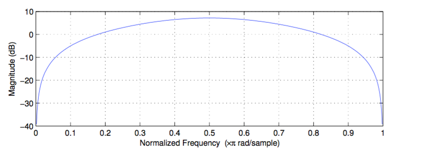# Classifying Discrete time filter

So let's say i have this frequency response of a digital filter:My question is how can i classify this type of filter ( low pass, high pass,...)?

It's a bandpass filter whose frequency response suppresses the low and high frequencies (with its zeros at $\omega = 0$ and $\omega = \pi$) and amplifies the band in between.
The bandwidth of the filter can be defined by reference to $-3_{dB}$ points, which would give you approximately $W_{-3dB} \approx 0.2\pi$, with a center frequency of $\omega_c = 0.5\pi$.
• So basically the high frequencies are $\omega =\pi$ and the low frequencies are $\omega=0$ or $\omega=2\pi$ ? – J. Barbosa May 31 '16 at 19:02
• in the discrete time domain, low frequencies are around $\omega = 2\pi k$ and high frequencies are around $\omega = \pi + 2\pi k$ for $k \in Z$ – Fat32 May 31 '16 at 19:06